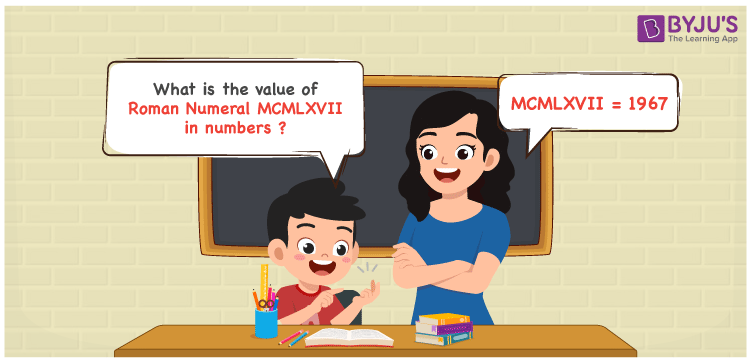Checkout JEE MAINS 2022 Question Paper Analysis : Checkout JEE MAINS 2022 Question Paper Analysis :

# MCMLXVII Roman Numerals

MCMLXVII Roman Numerals is 1967. Roman Numerals are the letters used by the ancient Romans for the representation of cardinal numbers. Students are advised to download Roman Numerals Conversion in PDF format to learn the conversion of Roman Numerals into numbers with speed and accuracy. Let us learn the simple tricks of expressing the number form of the Roman Numeral MCMLXVII in this article.

 Number Roman Numeral 1967 MCMLXVII

## How to Write MCMLXVII Roman Numerals?MCMLXVII Roman Numerals in numbers can be written by using any of the two methods given below

First Method:

• Break the Roman Numerals into single letters
• MCMLXVII = M + (M – C) + L + X + V + I + I
• Write the numerical value of each letter and add/subtract them
• MCMLXVII = 1000 + (1000 – 100) + 50 + 10 + 5 + 1 + 1 = 1967

Second Method:

In this method, we consider the groups of Roman Numerals for addition or subtraction

• MCMLXVII = M + CM + LX + VII = 1000 + 900 + 60 + 7 = 1967
• Therefore the numerical value of MCMLXVII Roman Numerals is 1967

## Video Lesson on Roman Numerals## Related Articles

Roman Numerals

Roman Numerals 1 to 50

Roman Numerals 1 to 100

Roman Numerals 1 to 1000

L Roman Numerals

Roman Numeral XXXIX

## Frequently Asked Questions on MCMLXVII Roman Numerals

### How do you write the numerical value of MCMLXVII Roman Numerals?

The numerical value of MCMLXVII Roman Numerals is written as 1967.

### What is the difference between 2000 and 1967 in Roman Numerals?

2000 – 1967 = 33. The number 33 in Roman Numerals is XXXIII. Hence the difference between 2000 and 1967 in Roman Numerals is XXXIII.

### Write the Arabic Numeral of MCMLXVII Roman Numeral.

1967 is the Arabic Numeral of MCMLXVII Roman Numeral.